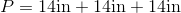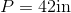# ISEE Middle Level Math : How to find the perimeter of a triangle

## Example Questions

### Example Question #21 : How To Find The Perimeter Of A Triangle

Use the following image to answer the question: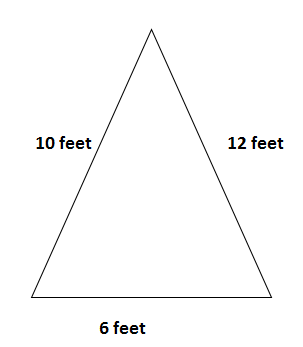Find the perimeter of the triangle.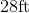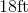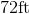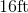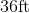Explanation:

To find the perimeter of a triangle, we will use the following formula: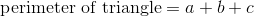where a, b, and c are the lengths of the sides of the triangle.

Now, let's look at the triangle.We can see the triangle has sides with lengths of 10 feet, 6 feet, and 12 feet.

Knowing this, we can substitute into the formula.  We get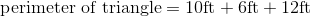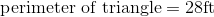### Example Question #22 : How To Find The Perimeter Of A Triangle

Find the perimeter of an equilateral triangle that has a base of 10 feet.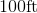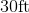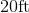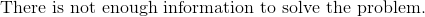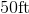Explanation:

To find the perimeter of a triangle, we will use the following formula:where a, b, and c are the lengths of the sides of the triangle.

Now, we know the base of the triangle is 10 feet.  Because it is an equilateral triangle, we know that all sides are equal.  Therefore, all sides are 10 feet.

Knowing this, we can substitute into the formula.  We get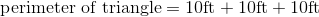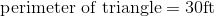### Example Question #23 : How To Find The Perimeter Of A Triangle

Find the perimeter of an equilateral triangle with a base of 6ft.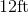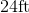Explanation:

To find the perimeter of a triangle, we will use the following formula:where a, b, and are the lengths of the sides of the triangle.

Now, we know the base of the triangle is 6ft.  Because it is an equilateral triangle, we know that all sides are equal.  Therefore, all sides are 6ft.

Knowing this, we can substitute into the formula.  We get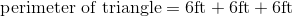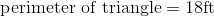### Example Question #24 : How To Find The Perimeter Of A Triangle

Find the perimeter of an equilateral triangle with a base of length 17in.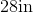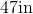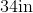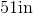Explanation:

To find the perimeter of a triangle, we will use the following formula:where a, b, and c are the lengths of the sides of the triangle.

Now, we know the base of the triangle has a length of 17in.  Because it is an equilateral triangle, all lengths are the same.  Therefore, all lengths are 17in.

Knowing this, we can substitute into the formula.  We get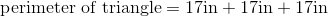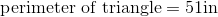### Example Question #25 : How To Find The Perimeter Of A Triangle

Find the perimeter of an equilateral triangle with a base of 14cm.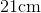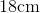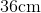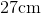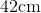Explanation:

To find the perimeter of a triangle, we will use the following formula:where a, b, and c are the lengths of the sides of the triangle.

Now, we know the base of the triangle is 14cm.  Because it is an equilateral triangle, all sides are equal.  Therefore, all sides are 14cm.

Knowing this, we can substitute into the formula.  We get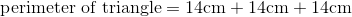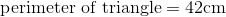### Example Question #26 : How To Find The Perimeter Of A Triangle

Find the perimeter of an equilateral triangle with a base of length 34in.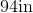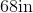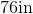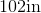Explanation:

To find the perimeter of a triangle, we will use the following formula: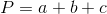where a, b, and c are the lengths of the sides of the triangle.

Now, we know the base of the triangle is 34in.  Because it is an equilateral triangle, all sides are equal.  Therefore, all sides are 34in.

Knowing this, we can substitute into the formula.  We get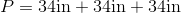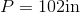### Example Question #27 : How To Find The Perimeter Of A Triangle

Find the perimeter of a triangle that has a base of 16in.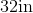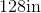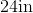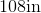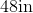Explanation:

To find the perimeter of a triangle, we will use the following formula:where a, b, and c are the lengths of the sides of the triangle.

Now, we know the base of the triangle is 16in.  Because it is an equilateral triangle, all sides are equal.  Therefore, all sides are 16in.

Knowing this, we will substitute into the formula.  We get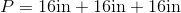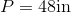### Example Question #28 : How To Find The Perimeter Of A Triangle

Use the following triangle to answer the question: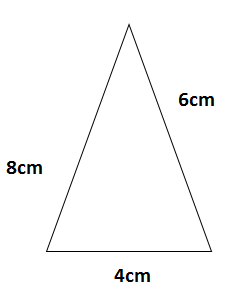Find the perimeter.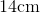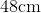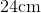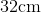Explanation:

To find the perimeter of a triangle, we will use the following formula:where a, b, and c are the lengths of the sides of the triangle.

Now, given the triangle,we can see it has sides with length 8cm, 4cm, and 6cm.  Knowing this, we can substitute into the formula.  We get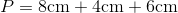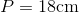### Example Question #29 : How To Find The Perimeter Of A Triangle

The perimeter of an equilateral triangle is 39cm.  Find the length of one side.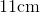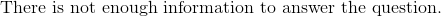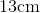Explanation:

To find the perimeter of an equilateral triangle, we use this formula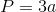where a is the length of one side.  An equilateral triangle has 3 equal sides.  That is why we multiply the length of one side by 3.  To find the length of one side, we will solve for a.

Now, we know the perimeter of the equilateral triangle is 39cm.  So, we can substitute.  We get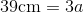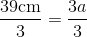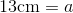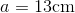Therefore, the length of one side of the equilateral triangle is 13cm.

### Example Question #30 : How To Find The Perimeter Of A Triangle

Find the perimeter of an equilateral triangle with a base of 14in.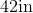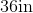Explanation:

To find the perimeter of a triangle, we will use the following formula:where a, b, and c are the lengths of the sides of the triangle.

Now, we know the base of the triangle is 14in.  Because it is an equilateral triangle, all sides are equal.  Therefore, all sides are 14in.  So, we can substitute.